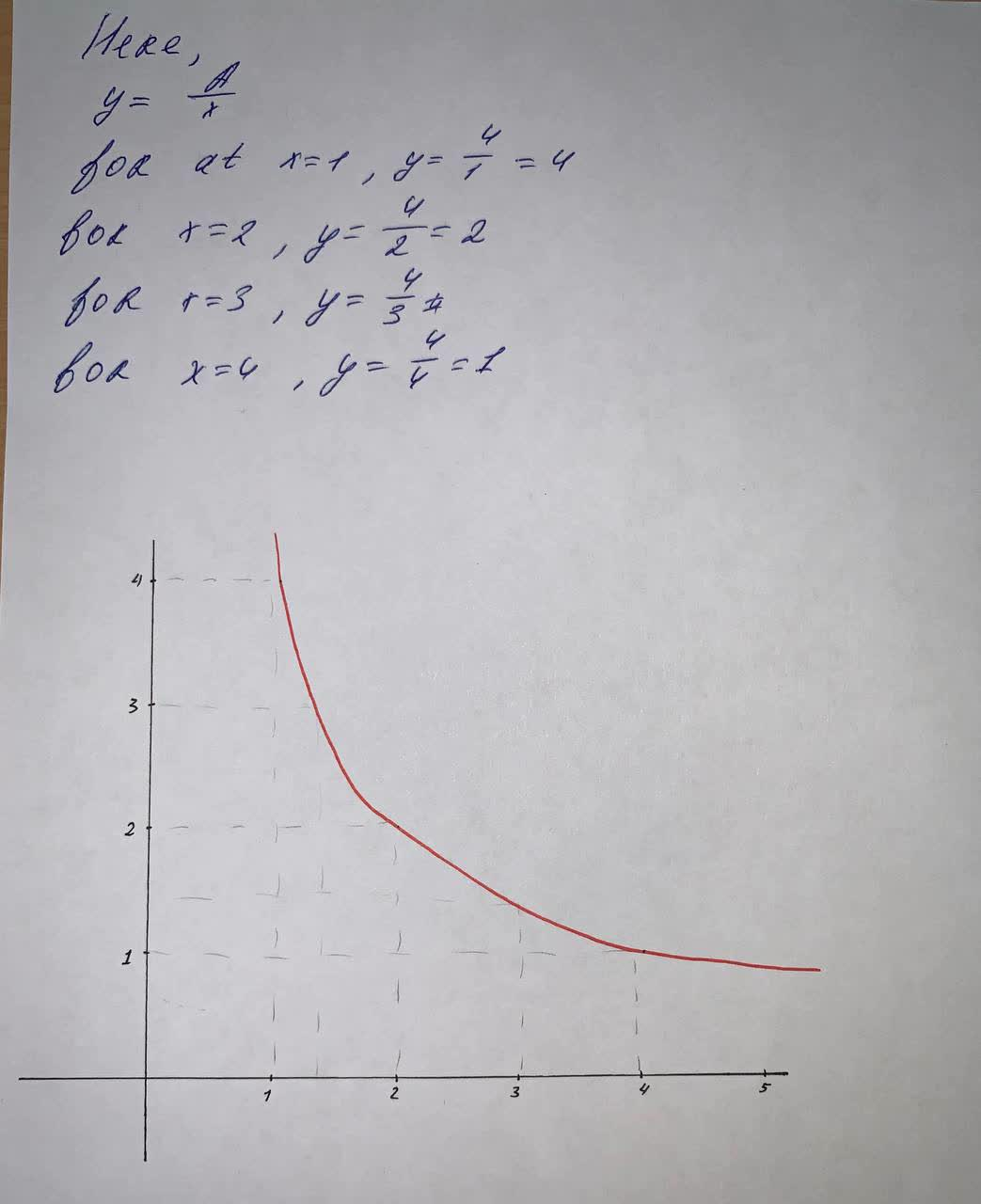#### Didn’t find what you are looking for?

Question# Consider the case when the constant A=4. Plot the graph of y=\frac{4}{x}

Linear equations and graphs
ANSWEREDConsider the case when the constant A=4. Plot the graph of $$y=\frac{4}{x}$$